Homework Help Question & Answers

# A rectangle is inscribed with its base on the x-axis and its upper corners on the...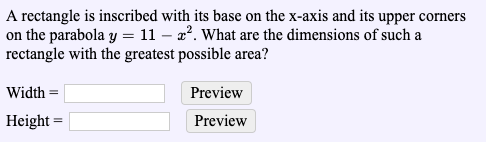A rectangle is inscribed with its base on the x-axis and its upper corners on the parabola y = 11-2P. What are the dimensions of such a rectangle with the greatest possible area? Width- Preview Height- Preview

#### Homework Answers

Answer #1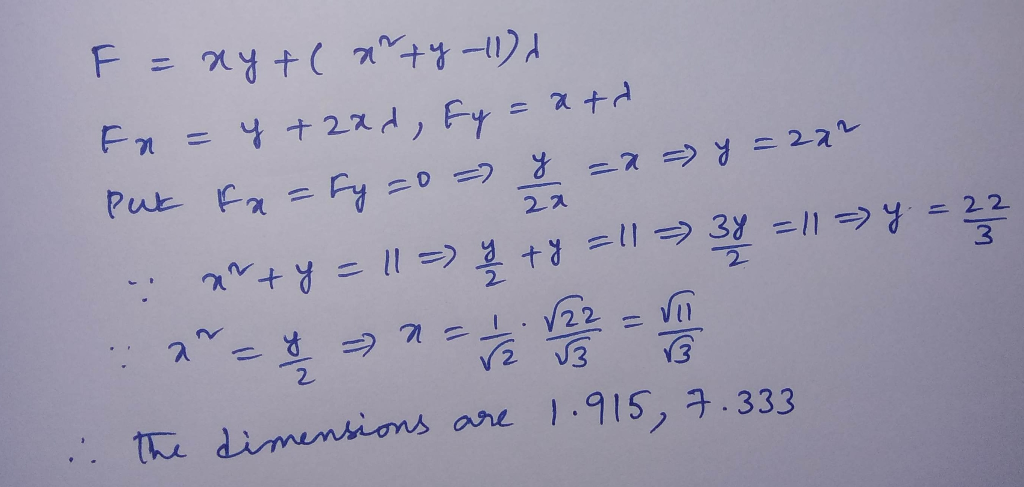Know the answer?
Your Answer:

#### Post as a guest

Your Name:

What's your source?

#### Earn Coin

Coins can be redeemed for fabulous gifts.

Not the answer you're looking for? Ask your own homework help question. Our experts will answer your question WITHIN MINUTES for Free.
Similar Homework Help Questions
• ### A rectangle is inscribed with its base on the x-axis and its upper corners on the parabola y=9-x^2

A rectangle is inscribed with its base on the x-axis and its upper corners on the parabola y=9-x^2. What are the dimensions of such a rectangle with the greatest possible area?

• ### Please do both 3. A rectangle has its base on the x-axis and it upper two...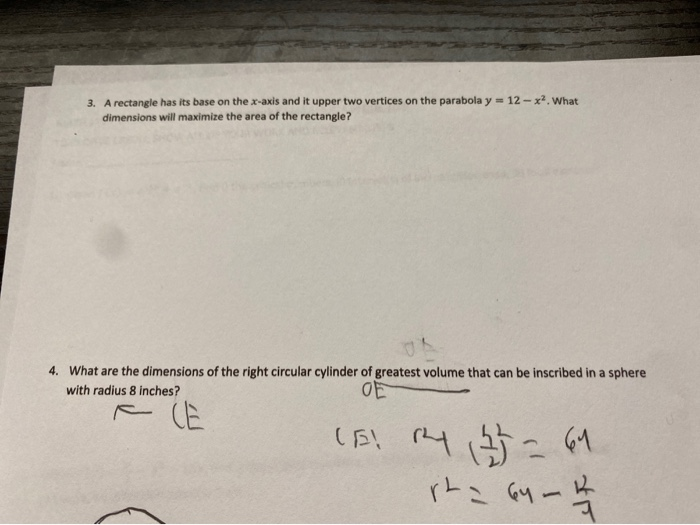Please do both 3. A rectangle has its base on the x-axis and it upper two vertices on the parabola y = 12 - x? What dimensions will maximize the area of the rectangle? 4. What are the dimensions of the right circular cylinder of greatest volume that can be inscribed in a sphere with radius 8 inches? OE (S! R4 135=61 rta 64 - 4

• ### 3. A rectangle has its base on the x-axis and it upper two vertices on the...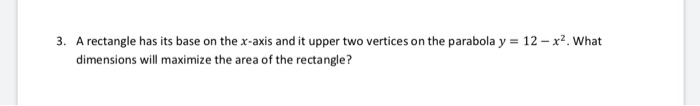3. A rectangle has its base on the x-axis and it upper two vertices on the parabola y = 12 - x2. What dimensions will maximize the area of the rectangle?

• ### A rectangle with its base on the x axis is to be inscribed under the positive portions of the graph of y=8-x^2

A rectangle with its base on the x axis is to be inscribed under the positive portions of the graph of y=8-x^2 . Write an equation for the area of the rectangle which uses only the variable x.

• ### If 2000 square centimeters of material is available to make a box with a square base...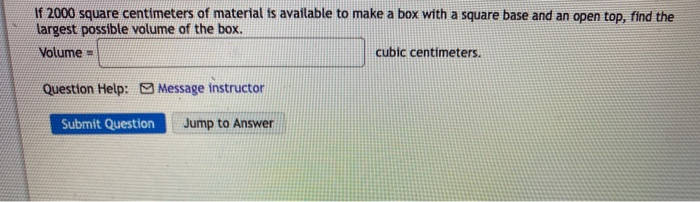If 2000 square centimeters of material is available to make a box with a square base and an open top, find the largest possible volume of the box. Volume cubic centimeters. Question Help: Message instructor Submit Question Jump to Answer A rectangle is inscribed with its base on the x-axis and its upper corners on the parabola y = 11 - 2?. What are the dimensions of such a rectangle with the greatest possible area? Width = Height = Question...

• ### Find the area of the largest rectangle that has its base on the x-axis and its other two vetices above the x-axis and lying on the parabola y=r-x^2

Find the area of the largest rectangle that has its base on the x-axis and its other two vetices above the x-axis and lying on the parabola y=r-x^2

• ### A baseball team plays in a stadium that holds 62000 spectators. With the ticket price at...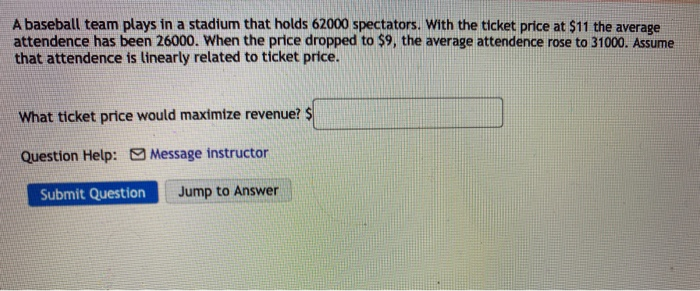A baseball team plays in a stadium that holds 62000 spectators. With the ticket price at \$11 the average attendence has been 26000. When the price dropped to \$9, the average attendence rose to 31000. Assume that attendence is linearly related to ticket price. What ticket price would maximize revenue? \$ Question Help: Message instructor Submit Question Jump to Answer A farmer finds that if she plants 40 trees per acre, each tree will yield 20 bushels of fruit. She...

• ### Use the method of Lagrange multipliers to find the dimensions of the rectangle of greatest area...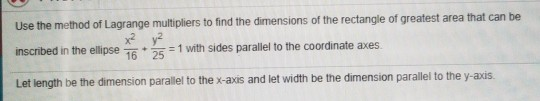Use the method of Lagrange multipliers to find the dimensions of the rectangle of greatest area that can be inscribed in the ellipse = 1 with sides parallel to the coordinate axes Let length be the dimension parallel to the x-axis and let width be the dimension parallel to the y-acis.

• ### A given rectangle is inscribed in the parabola as shown here. a. Find the coordinates of...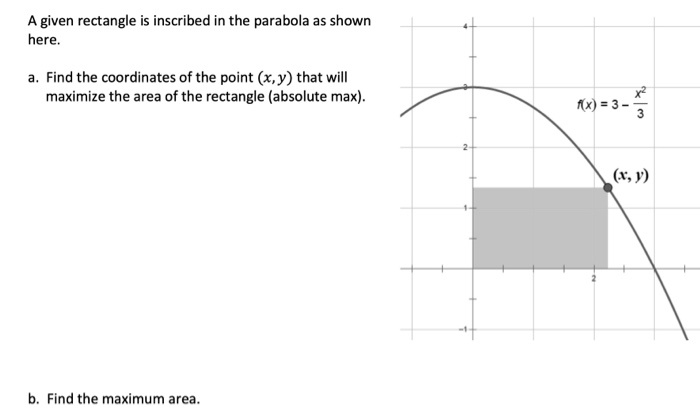A given rectangle is inscribed in the parabola as shown here. a. Find the coordinates of the point (x,y) that will maximize the area of the rectangle (absolute max). (x, y) b. Find the maximum area.

• ### Show that if a rectangle has its base on the x-axis and two of its vertices on the curve y = e^-x^2 , then the rectangle will have the largest possible area when the two vertices are at the points of inflection of the curve

Show that if a rectangle has its base on the x-axis and two of its vertices on the curve y = e^-x^2 , then the rectangle will have the largest possible area when the two vertices are at the points of inflection of the curve.

Free Homework App

Scan Your Homework
to Get Instant Free Answers
Need Online Homework Help?

Get Answers For Free
Most questions answered within 3 hours.